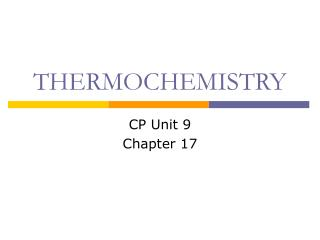DownloadDownload PresentationTHERMOCHEMISTRY

# THERMOCHEMISTRY

Télécharger la présentation## THERMOCHEMISTRY

- - - - - - - - - - - - - - - - - - - - - - - - - - - E N D - - - - - - - - - - - - - - - - - - - - - - - - - - -
##### Presentation Transcript

1. THERMOCHEMISTRY CP Unit 9 Chapter 17

2. Thermochemistry 17.1 • Thermochemistry is the study of energy changes (HEAT) that occur during chemical reactions and changes in state.

3. ENTHALPY 17.2 • Enthalpy = a type of chemical energy, sometimes referred to as “heat content”, ΔH (the heat of reaction for a chemical reaction) • endothermic reactions (feels cold): • q = ΔH > 0 (positive values) • exothermic reactions (feels hot): • q (heat) = ΔH (enthaply, heat of rxn) < 0 (negative values)

4. Endo vs. Exo- Endothermic reactions: absorbs heat from surroundings (+). • If you touch an endothermic reaction it feels COLD Exothermic reactions: release heat to the surroundings (-) • If you touch an exothermic reaction it feels HOT

5. Magnitude of Heat Flow • Units of heat energy: • 1 kcal = 1,000 cal = 1 Cal (nutritional) • 1 kJ = 1,000 J • 1 calorie = 4.184 J • 1 kcal = 4.184 kJ

6. Thermochemical Equations • A chemical equation that shows the enthalpy (H) is a thermochemical equation.

7. Rule #1 The magnitude (value) of H is directly proportional to the amount of reactant or product. H2 + Cl22HCl H = - 185 kJ * meaning there are 185 kJ of energy RELEASED for every: 1 mol H2 1 mol Cl2 2 moles HCl

8. Rules of Thermochemistry Example 1: H2 + Cl22HCl H = - 185 kJ Calculate H when 2.00 moles of Cl2 reacts.

9. Rules of Thermochemistry Example 2: Methanol burns to produce carbon dioxide and water: 2CH3OH + 3O2 2CO2 + 4H2O H = - 1454 kJ What mass of methanol is needed to produce 1820 kJ?

10. Rule #2 H for a reaction is equal in the magnitude but opposite in sign to H for the reverse reaction. (If 6.00 kJ of heat absorbed when a mole of ice melts, then 6.00 kJ of heat is given off when 1.00 mol of liquid water freezes)

11. Rules of Thermochemistry Example 1: Given: H2 + ½O2 H2O H = -285.8 kJ Reverse: H2O  H2 + ½O2H = +285.8 kJ

12. Example 2 CaCO3 (s)  CaO (s) + CO2 (g) H = 178 kJ What is the H for the REVERSE RXN? CaO (s) + CO2 (g)  CaCO3 (s) H = ?

13. Alternate form of thermochem. eq. • Putting the heat content of a reaction INTO the actual thermochemical eq. • H2 + ½O2 H2O H = -285.8 kJ Exothermic (H is negative) Heat is RELEASED as a PRODUCT The alternate form is this:H2 + ½O2 H2O +285.8 kJ

14. EX: 2 NaHCO3 + 129 kJ  Na2CO3 + H2O + CO2 Put in the alternate form The alternate form is this: 2 NaHCO3 Na2CO3 + H2O + CO2H =+ 129 kJ

15. Put the following in alternate form • H2 + Cl2 2 HCl H = -185 kJ • 2 Mg + O2 2 MgO + 72.3 kJ • 2 HgO  2 Hg + O2H = 181.66 kJ

16. Enthalpies of Formation standard conditions enthalpy change (delta) formation

17. Enthalpies of Formation • usually exothermic • see table for Hf value (Table A3) • enthalpy of formation of an element in its stable state = 0 • these can be used to calculate H for a reaction

18. Standard Enthalpy Change Standard enthalpy change, H, for a given thermochemical equation is = to the sum of the standard enthalpies of formation of the product – the standard enthalpies of formation of the reactants. sum of (sigma)

19. Standard Enthalpy Change • elements in their standard states can be omitted: 2 Al(s) + Fe2O3(s) 2 Fe(s) + Al2O3(s) ΔHrxn = (ΔHfproducts) - (ΔHfreactants) ΔHrxn = ΔHfAl2O3 - ΔHfFe2O3 ΔHrxn = (-1676.0 kJ) – (-822.1 kJ) ΔHrxn = -853.9 kJ

20. Standard Enthalpy Change • the coefficient of the products and reactants in the thermochemical equation must be taken into account: O2(g) + 2SO2 (g) 2SO3 (g) ΔHrxn = (ΔHfproducts) - (ΔHfreactants) ΔHrxn = 2ΔHfSO3 - 2ΔHfSO2 ΔHrxn = 2(-395.7 kJ) – 2(-296.8 kJ) ΔHrxn = -197.8 kJ

21. Standard Enthalpy Change - Calculate the standard heat for formation of benzene, C6H6, given the following thermochemical equation: Don’t forget the coefficents! C6H6(l) + 15/2 O2(g)  6CO2(g) + 3H2O(l)H = -3267.4 kJ -393.5kJ -285.8kJ Total X -3267.4kJ = [6(-393.5kJ)+3(-285.8kJ)]–X -3267.4kJ = -3218.4–X -49.0kJ = – X X = +49.0 kJ